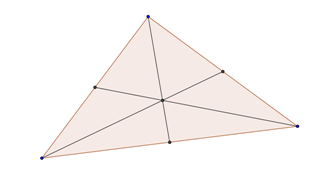The centroid of a triangle is located at the intersection of the medians of the triangle. Depending on context, this point is also known as the centre of mass or centre of gravity of the triangle. If you make a model of the triangle out of some flat, rigid material, the centroid is the point at which you can balance it on a point: for any line through the centroid, the total torque produced about that line by gravity is zero.

Taking that last sentence as the definition of "centroid" for any two-dimensional shape, every such shape has a centroid. (I am handwaving a bit about the definition of "shape" here.) Here is a very rough sketch of a proof. For each point in the shape, consider all lines through that point and look at the biggest net torque produced by the action of gravity on the shape. Find a point that minimizes this number. The number there must be zero, because otherwise you can decrease it by moving perpendicular to a line through that point that gives the maximum torque.

The centroid is the "mean position" of all points in the shape, in the following sense: it equals the integral of (position) d(area) over the whole shape, divided by the integral of 1 d(area). (Imagine dividing the shape into many tiny regions of equal area and averaging their locations. Or dividing it into many tiny regions of not-necessarily-equal area, and averaging their locations weighted by their areas.)

Shapes in more than two dimensions also have centroids.